# KINEMATICS

## KINEMATICS

Inertial frame of reference:  Reference frame in which Newtonian mechanics holds are called inertial reference frames or inertial frames. The reference frame in which Newtonian mechanics does not hold is called non-inertial reference frames or non-inertial frames.

The average speed vav and average velocity  of a body during a time interval? t is defined as,

vav= average speed

ntent -->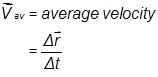=? s/? t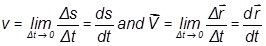Instantaneous speed and velocity are defined at a particular instant and are given by

## Note: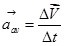(a) A change in either speed or direction of motion results in a change in velocity

(b) A particle which completes one revolution, along a circular path, with uniform speed is said to possess zero velocity and non-zero speed.

(c) a particle can’t possess zero speed with a non-zero velocity.

Average acceleration is defined as the change in velocity  over a time interval? t.

The instantaneous acceleration of a particle is the rate at which its velocity is changing at that instant.

The three equations of motion for an object with constant acceleration are given below.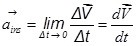(a) v= u+at

(b) s= ut+1/2 at2

(c) v2=u2+2as

Here u is the initial velocity, v is the final velocity, a is the acceleration, s is the displacement traveled by the body and t is the time.

Note: Take the ‘+ve’ sign for a when the body accelerates and takes ‘–ve’ sign when the body decelerates.

The displacement by the body in nth second is given by,

snu + a/2 (2n-1)

Position-time (x vs t), velocity-time (v vs t) and acceleration-time (a vs t) graph for motion in one-dimension:

(I) Variation of displacement (x), velocity (v), and acceleration (a) to time for different types of motion.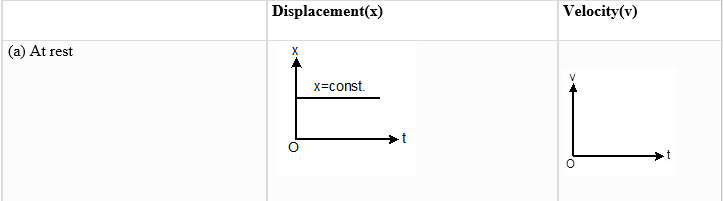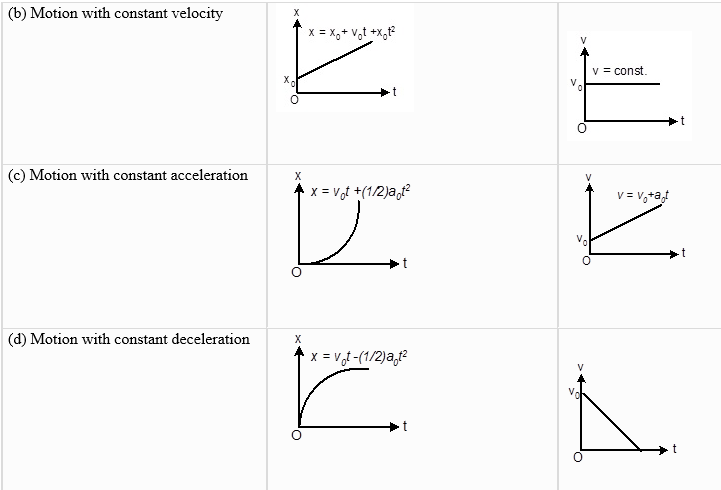## Scalar Quantities:

A scale or scalar sum of physics is often followed by units of measurement, may be represented by a single aspect of a number field such as a real number. A scalar is typically a physical sum of magnitude, probably a symbol, and no other properties. (e.g-mass, length, volume, density)

## Vector Quantities:

Vector quantities are those quantities that require magnitude as well as direction for their complete specification. (e.g-displacement, velocity, acceleration, force)

## Null Vector (Zero Vectors):

It is a vector having zero magnitudes and an arbitrary direction.

When a null vector is added or subtracted from a given vector the resultant vector is the same as the given vector.

The dot product of a null vector with any arbitrary is always zero. Cross product of a null vector with any other vector is also a null vector.

# Collinear vector:

Vectors having a common line of action are called a collinear vector. There are two types.

Parallel vector (θ=0°):

Two vectors acting along the same direction are called parallel vectors.

Anti-parallel vector (θ=180°): Two vectors which are directed in opposite directions are called anti-parallel vectors.

Co-planar vectors- Vectors situated in one plane, irrespective of their directions, are known as co-planar vectors.

Vector addition is commutative-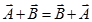Vector addition is associative-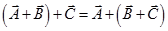Vector addition is distributive-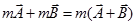## Triangles Law of Vector addition:

If two vectors are represented by two sides of a triangle, taken in the same order, then their resultant is represented by the third side of the triangle taken in the opposite order.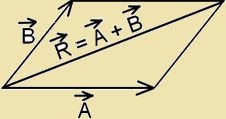The magnitude of the resultant vector:-

R=√(A2+B2+2ABcosθ)

Here θ is the angle between and .

If β is the angle between  and,

then,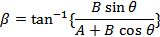If three vectors acting simultaneously on a particle can be represented by the three sides of a triangle taken in the same order, then the particle will remain in equilibrium.

So,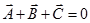## Parallelogram law of vector addition: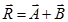R=√(A2+B2+2ABcosθ),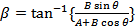## Cases 1:

When, θ=0°, then,

RA+B (maximum), β=0°

## Cases 2:

When, θ=180°, then,

RAB (minimum), β=0°

## Cases 3:

When, θ=90°, then,

R=√(A2+B2), β = tan-1 (B/A)

The process of subtracting one vector from another is equivalent to adding, vectorially, the negative of the vector to be subtracted.

So,

## Resolution of a vector in a plane: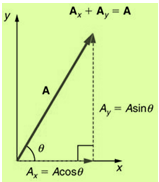Product of two vectors:

## (a) Dot product or scalar product: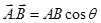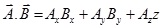Here A is the magnitude of , B is the magnitude of  and θ is the angle between  and .

(i) Perpendicular vector: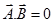(ii) Collinear vector: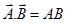When Parallel vector (θ=0°),

When Antiparallel vector (θ=180°),

## (b) Cross product or Vector product: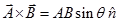Or,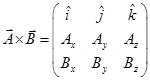Here A is the magnitude of , B is the magnitude of ,θ is the angle between  and/and  is the unit vector in a direction perpendicular to the plane containing  and .

(i) Perpendicular vector (θ=90°):

(ii) Collinear vector:-

When, Parallel vector (θ=0°),(null vector)

When, θ=180°,(null vector)

## Unit Vector:

The unit vector of any vector is a vector having a unit magnitude, drawn in the direction of the given vector.

In three dimensions,

## Area:

Area of triangle:

Area of parallelogram:

Volume of parallelepiped:

## Equation of Motion in an Inclined Plane:

Perpendicular vector: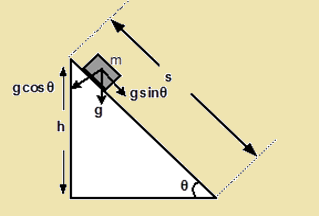At the top of the inclined plane (t = 0, u = 0 and a = g cinq  ), the equation of motion will be,

(a) v= (g sinθ)t

(b) s = ½ (g sinθ) t2

(c) v= 2(g sinθ)s

(ii) If the time taken by the body to reach the bottom is t, then   s = ½ (sinθt2

= √(2s/sinθ)

But sinθ =h/s   or sh/sinθ

So, =(1/sinθ) √(2h/g)

(iii) The velocity of the body at the bottom

v=g(sinθ)t

=√2gh

The relative velocity of object A  to object B is given by

VAB=VAVB

Here, Vis called reference object velocity.

Variation of mass:- Following Einstein’s mass-variation formula, the relativistic mass of the body is defined as,

mm0/√(1-v2/c2)

Here, mis the rest mass of the body, v is the speed of the body and c is the speed of light.

Projectile motion in a plane:- If a particle having initial speed u is projected at an angle θ (angle of projection) with the x-axis, then,

Time of Flight, = (2u sinα)/g

Horizontal Range, u2sin2α/g

Maximum Height, u2sin2α/2g

Equation of trajectory, xtanα-(gx2/2u2cos2α)

## The motion of a ball:

• When dropped:

Period, t=√(2h/g) and speed, v=√(2gh

• When thrown up:

Time period, t=u/g and height, h = u2/2g

## Condition of equilibrium:

(a)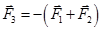(b) |F1+F2|≥|F3|≥| F1-F2|

#### Related Posts